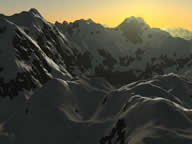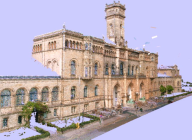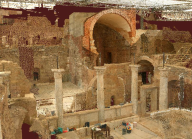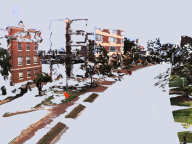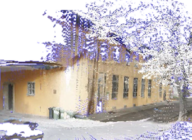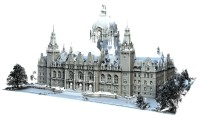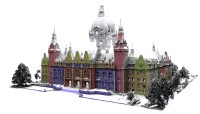A | B | C | D | E | F
G | H | I | J | K | L | M
N | O | P | Q | R | S | T
U | V | W | X | Y | Z
max planck institut
informatik# Lecture

Statistical Geometry Processing (Winter Semester 2011/2012)

# Statistical Geometry Processing

This lecture gives an introduction to the research field of statistical geometry processing. Informally spoken, the goal in this research area is to develop algorithms that are able to understand geometry. We want to develop algorithms that can analyze and represent the structure of geometric objects, and use this knowledge for analyzing, reconstructing, and modeling 3D geometry. There are a many application domains where such approaches are needed, such as medical data analysis (3D tomography), 3D scanning of cities for virtual maps, or content creation for 3D computer games.

The approach of this lecture will be to look at mathematical tools and then see how these could be applied: Figuratively spoken, we will iterate between searching for hammers (tools) and nails (problems to be solved). We will look at the theory, the applications, and conduct exercises to test the tools in practice.

Many of the techniques introduced in this lecture are actually rather generic and applicable beyond the domain of computer graphics and vision so that the lecture might be useful even for solving problems in other areas of algorithmic data analysis.

This is the first time this lecture is held. Therefore, the information given is so far still subject to change.

# Lecture Topics

The topics covered in this lecture will probably include (subject to change):

Mathematical background:

• Differential geometry
• Basic probability theory & statistics (recap)
• Calculus of variation
• Local and global optimization techniques

Machine learning fundamentals

• Introduction to statistical problem modeling

Modeling tools

• Least-squares fitting (regression, total least-squares, moving least-squares)
• Robust statistics
• Linear and non-linear principal component analysis and dimensionality reduction
• Linear and non-linear shape spaces

Surface reconstruction

• Surface reconstruction approaches

Correspondence problems

• Rigid shape matching
• Non-rigid shape matching
• Local and global optimization techniques
• Invariant features (rigid motions / isometries / semantic classes)
• Invariant descriptors
• Intrinsic geometry for non-rigid shape matching
• Robustness issues (noise, incomplete data, outliers)
• Machine learning techniques: semantic matching

# Prerequisites

The course is targeting master students and advanced bachelor students (towards the end of their studies). We will introduce most of the necessary background in the course, but some prior knowledge in the following areas might still be required to follow at the intended pace:

• Basic math (calculus, linear algebra, basic probability theory)
• Some prior exposure to computer graphics (basic modeling techniques, 3D geometry)
• Prior experience with computer vision / machine learning is a plus, but not required.

# Location

The lecture will be held Tuesdays 16-18h (s.t.) in E1 4 (MPI-Informatik), Room 024 (ground floor).

Excercise course: Wednesday, 12:00-13:30h (s.t.) in E1 4 (MPI-Informatik), Room t.b.a..

# Organization

There will be a lecture (2h a week) and exercises (2h a week). The exercises will focus on practical experiments with the techniques discussed in the lecture.

If you are interested in the course, and/or you have any questions or suggestions relating the course, feel invited to contact us via email at any time.

# Slides

This is the collection of slides available so far. The schedule for future slides is only approximate. You need a password to access the slides; for external viewers, a public version is provided below as well.

Public versions of the slides (only minor differences, no password required):

# Assignments

Here is the collection of assignment sheets handed-out so far.

# Software Framework

 The GeoX framework: Portable source code (Last update: Oct. 25th 2011) Tested with Microsoft VS 2008, VS 2010 on Windows and GCC/qmake on Linux. Short Introduction: Using the GeoX Framework (Last update: Oct. 25th 2011) Tutorial: Assignment #0 (see also above) Update #1: source code (Last update: Nov. 2nd 2011) New version supports SVD via OpenCV New image viewer (as described in Assignment #0) Currently only for Visual Studio 2010 (contact us for other platforms)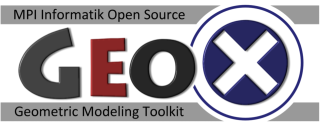# Schedule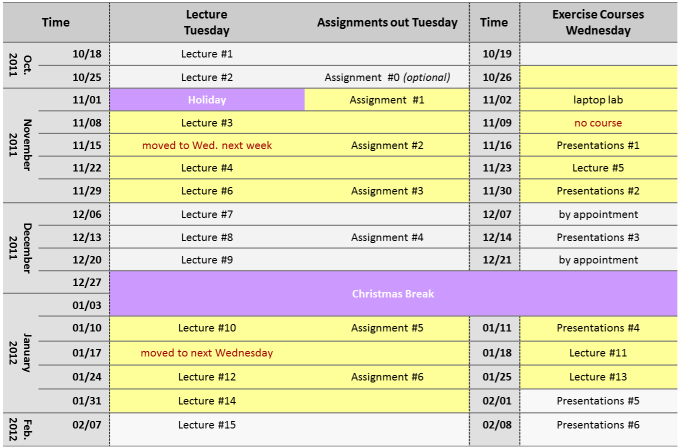(Schedule updated: Jan. 30th 2012, 19:07h CET)